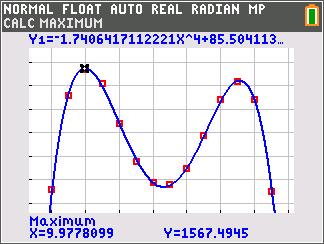•##### Download

•• ##### Device
• TI-84 Plus
• TI-84 Plus Silver Edition
•TI-84 Plus C Silver Edition
•TI-84 Plus CE
• ##### Software

TI Connect™ CE

# Precalculus: Ride the Rollercoaster

by Texas Instruments#### Overview

Students use polynomial regression to develop and assess the fit of equations modeling data. The equation models are then evaluated for reasonableness in their use for extrapolating beyond the given data sets.

#### Key Steps

•In this activity, students use polynomial regression to develop and assess the fit of equations modeling data. The equation models are then evaluated for reasonableness in their use for extrapolating beyond the given data sets.

•Students will learn to assess the fit of an equation to a set of data using R2. They learn that at times, the equation that best fits may not be what they first thought. Visual assessment may lead the student to believe that a cubic equation will fit best, while the quartic equation fits slightly better and yields an R2 value closer to 1.

•In the additional problems, students will create a scatter plot and use polynomial regression to find an appropriate equation to fit the given NASDAQ index data and weekly gasoline price data.